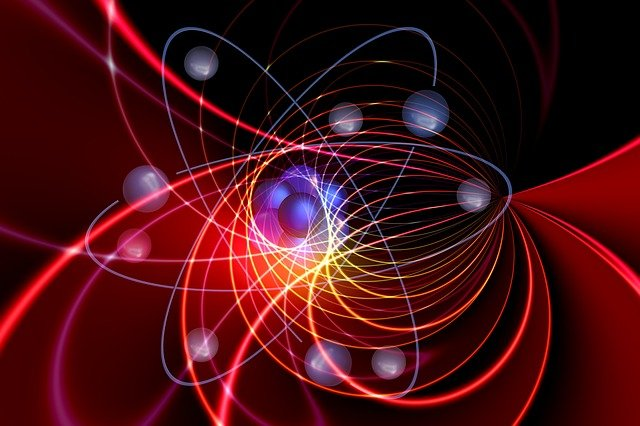In the classical formulation of Quantum Mechanics, called Quantum Sharp Measurement, it is assumed that physical measurements are always sharp. In other words, we assume that our apparatus used in the experiment gives us accurate readings of the measurements, for instance, of a particle’s position or momentum. However in the real world, experiments involve inaccurate measurements by nature, therefore we often need to work in the more general framework called Quantum Unsharp Measurement. In this unsharp setting, the notion of a quantum event has to be extended.The mathematical theory of this has been very well developed during the twentieth century by pioneers such as John von Neumann, Eugen Paul Wigner, Paul Dirac, etc. This theory builds on a subfield of Mathematics called Operator Theory, where quantum sharp events correspond to so-called projection operators, and they are equipped with three important relations.

The first one is called ortho-complementation, which expresses that two quantum sharp events are each other’s negations, that is, one happens exactly when the other does not. The second relation is the so-called Loewner order, we say that one quantum sharp event is less than or equal to the other if the occurrence of the first one always implies the other’s occurrence. The third relation is called coexistence, where two quantum events are said to be coexistent with each other if they can be measured together by applying a suitable apparatus. For instance, the quantum spin of a particle cannot be measured in two different directions at the same time (unless these directions are parallel). All these three relations are mathematically well-understood in the Quantum Sharp Measurement setting.

In reality measurements are inaccurate by nature, therefore we often need to work in the more general framework called Quantum Unsharp Measurement. Roughly speaking, we can define a general quantum unsharp event as a weighted average of quantum sharp events. In this setting we also have the above mentioned three relations: ortho-complementation, Loewner order, and coexistence (joint measurability). The mathematical formulation of the first two is very similar to the sharp setting and hence well-understood. However, interestingly, the mathematical formulation of coexistence becomes much more complicated. In particular, certain basic questions which are easy to answer in the Quantum Sharp Measurement setting become very difficult questions in the Quantum Unsharp Measurement setting. For instance, one such question is to decide when two quantum events are coexistent with exactly the same quantum events? This question in the sharp setting is a rather straightforward exercise in an undergraduate course. However, the question in the unsharp setting is much more involved, and it was our paper’s first main achievement. By answering this question in the unsharp setting, we hope to deepen the understanding of this interesting relation and influence further research on this topic in Quantum Mechanics and Quantum Information Theory.

The other main achievement of our paper is about so-called quantum mechanical symmetry transformations. These are transformations that leave a certain quantity or relation unchanged when applied to the quantum system. Such symmetry transformations are important because they provide valuable tools in the examination of the evolution of certain quantum systems. The most classical example of this is probably Wigner’s theorem on quantum mechanical symmetry transformations, which is a vital tool in obtaining the general time-dependent Schroedinger equation, by purely mathematical means. The symmetry transformations of quantum sharp events are quite well-understood in the literature. In the unsharp setting however this is not so. In our paper we characterised all symmetry transformations of quantum unsharp events with respect to coexistence. We hope that this result will help to understand the evolution of quantum systems better in the Quantum Unsharp Measurement setting.

Gyorgy Geher is an Leverhulme Fellow in the Department of Mathematics and Stastitics and was awarded the Research Output Prize for Early Career Researchers 2021 for the Environment research theme.

The prize was awarded for his article, ‘Coexistency on Hilbert Space Effect Algebras and a Characterisation of Its Symmetry Transformations’ in Communications in Mathematical Physics 379, 1077–1112 (2020).